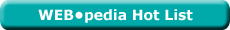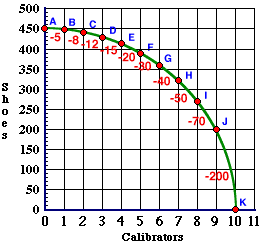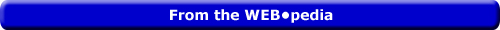Friday  March 31, 2023
 AmosWEB means Economics with a Touch of Whimsy!YTM: The common abbreviation for yield to maturity, which is the annual rate of return on a financial asset that is held until maturity. Yield to maturity depends on both the coupon rate and the face or par value paid at maturity. If the selling price of a financial asset is equal to its par value, then the yield to maturity is equal to the current yield and the coupon rate. However, if the asset is selling at a discount, then the yield to maturity exceeds the current yield, which is greater than the coupon rate. And if the asset is selling at a premium, then the yield to maturity is less than the current yield, which is below than the coupon rate.Most Viewed (Number) Visit the WEB*pediaLesson 4: Production Possibilities | Unit 3: The Curve Page: 11 of 24

 Topic: Slope and Cost <=PAGE BACK | PAGE NEXT=>The slope of a line is measured by calculating the change in the value measured on the vertical axis divided by the change in the value measured on the horizontal axis.
• Another way of saying this is to take the rise over the run.

 rise change in shoes slope = ---- = -------------------------- run change in calibrators

• Slope between I and J is -70. Rise is a decrease of 70. Run is an increase of 1.
The slope of the production possibilities curve is the opportunity cost of the good measured on the horizontal axis, which in our example is clock calibrators.

| | |INCOME EFFECT

The change in quantity demanded that results because a change in the demand price of a good affects real income (that is, the purchasing power of income) even though nominal income remains the same. This is one of two reasons, or effects, underlying the law of demand and the negative slope of the market demand curve. The other is the substitution effect.WHITE GULLIBON[What's This?] Today, you are likely to spend a great deal of time watching infomercials trying to buy either a wall poster commemorating the first day of winter or blue cotton balls. Be on the lookout for malfunctioning pocket calculators.Your Complete ScopeNatural gas has no odor. The smell is added artificially so that leaks can be detected."A leader, once convinced that a particular course of action is the right one, must . . . be undaunted when the going gets tough."-- President Ronald ReaganSURSeemingly Unrelated RegressionsA PEDestrian's Guide Xtra CreditTell us what you think about AmosWEB. Like what you see? Have suggestions for improvements? Let us know. Click the User Feedback link.| | | | | | | | | | |
| | | |

Thanks for visiting AmosWEB